## One circle has a circumference half as large as that of a second circle. Which ratio compares the diameter of the first circle t

Question

One circle has a circumference half as large as that of a
second circle. Which ratio compares the diameter of the
first circle to the diameter of the second circle?

in progress 0
6 months 2021-07-25T03:20:56+00:00 1 Answers 29 views 0

The ratio that compares the diameter of the first circle to the diameter of the second circle isStep-by-step explanation:

Circumference of a circle:

The circumference of a circle of diameter d is given by: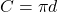In which d is the diameter.

One circle has a circumference half as large as that of a second circle.

We have that: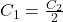So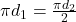Simplifying by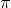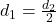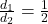The ratio that compares the diameter of the first circle to the diameter of the second circle is